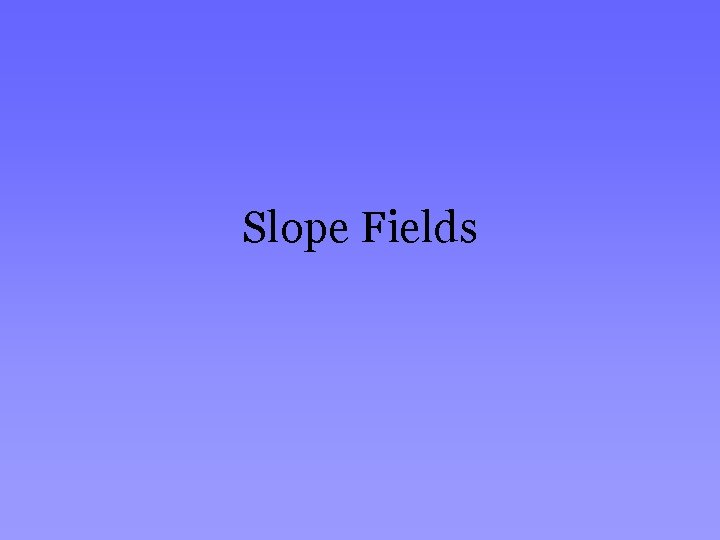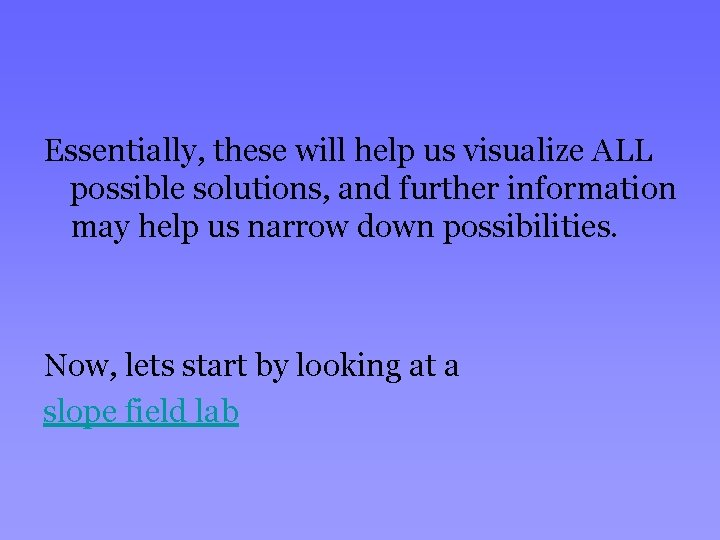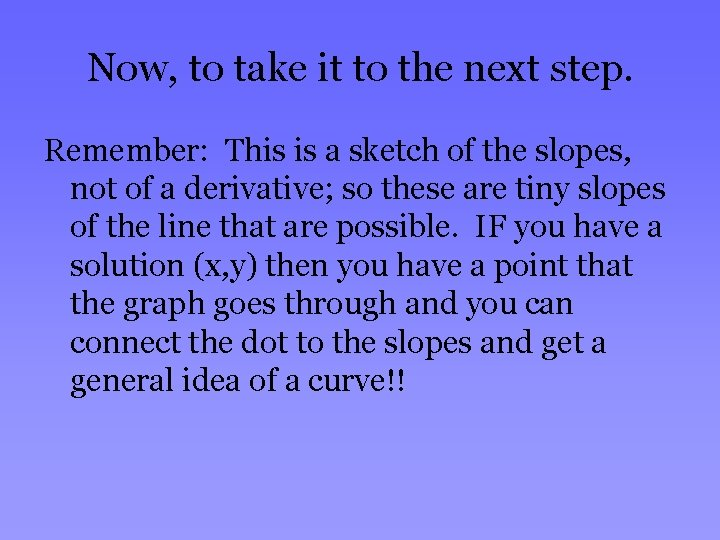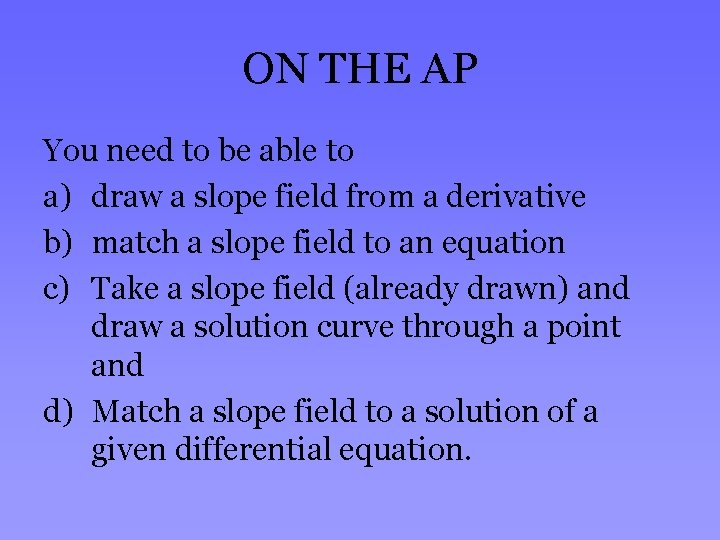# Slope Fields What are Slope Fields Slope Fields

• Slides: 6Slope FieldsWhat are Slope Fields? Slope Fields are a graphical method for solving differential equations that may not be solvable by traditional methods we’ve covered so far. From a derivative we can sketch a picture of all the slopes possible from the given differential equation. If we then have some initial information we can draw a pretty accurate sketch.Essentially, these will help us visualize ALL possible solutions, and further information may help us narrow down possibilities. Now, lets start by looking at a slope field labWhat did we see? If you know the differential equation, you simply draw little lines representing the slope at all x/y pairs within a certain area. It is VERY tedious, and that’s why they often use calculators to do the work. BUT, if we know how to do this, we can certainly go backwards.Now, to take it to the next step. Remember: This is a sketch of the slopes, not of a derivative; so these are tiny slopes of the line that are possible. IF you have a solution (x, y) then you have a point that the graph goes through and you can connect the dot to the slopes and get a general idea of a curve!!ON THE AP You need to be able to a) draw a slope field from a derivative b) match a slope field to an equation c) Take a slope field (already drawn) and draw a solution curve through a point and d) Match a slope field to a solution of a given differential equation.